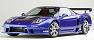Release Date: Jul 24, 2009
•#25
Nekem a kedvencem VÉKOND.Az amerikai kormány állatokat képez ki titkos akciókra. Az állatcsapat most hörcsögökből, vakondból és egy légyből áll. Ez a szupercsapat a Fantasztikus Négyes-ekre, sőt még James Bondra is ráver.

he g-force (pronounced /ˈdʒiː/) experienced by an object is its acceleration relative to free-fall.[not in citation given] The term g-force is considered a misnomer, as g-force is not a force but an acceleration.

The unit of measure used is the g- the acceleration due to gravity at the Earth's surface and it can be written g, g, or G. The unit g is not one of the SI International System of Units which uses 'g' for gram, and "G" could be confused with the standard symbol for the gravitational constant, but they are both distinct. The SI unit of acceleration is m/s2. However, objects experiencing g-forces are not necessarily changing velocity or position, so standard units of acceleration, which do not necessarily require stress in free fall, are by convention not used to express g-force.

* The g-force acting on a stationary object resting on the Earth's surface is 1 g (upwards) and results from the resisting reaction of the Earth's surface bearing upwards equal to an acceleration of 1 g, and is equal and opposite to standard gravity (symbol: gn), defined as 9.80665 metres per second squared.
* The g-force acting on an object in any weightless environment such as free-fall in a vacuum is 0 g.
* The g-force acting on an object under acceleration can be much greater than 1 g, for example, the dragster pictured right can exert a horizontal g-force of 5.3 when accelerating.
* The g-force acting on an object under acceleration downwards can be negative, for example when fully inverted in a rollercoaster loop.

Measurement of g-force is typically achieved using an accelerometer (see discussion below in Measuring g-force using an accelerometer). In certain cases g-forces may be measured using suitably calibrated scales.